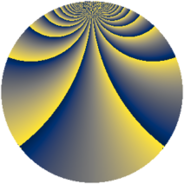# Properties

 Label 2760.2.bqLevel $2760$ Weight $2$ Character orbit 2760.bq Rep. character $\chi_{2760}(1243,\cdot)$ Character field $\Q(\zeta_{4})$ Dimension $528$ Sturm bound $1152$

# Related objects

## Defining parameters

 Level: $$N$$ $$=$$ $$2760 = 2^{3} \cdot 3 \cdot 5 \cdot 23$$ Weight: $$k$$ $$=$$ $$2$$ Character orbit: $$[\chi]$$ $$=$$ 2760.bq (of order $$4$$ and degree $$2$$) Character conductor: $$\operatorname{cond}(\chi)$$ $$=$$ $$40$$ Character field: $$\Q(i)$$ Sturm bound: $$1152$$

## Dimensions

The following table gives the dimensions of various subspaces of $$M_{2}(2760, [\chi])$$.

Total New Old
Modular forms 1168 528 640
Cusp forms 1136 528 608
Eisenstein series 32 0 32

## Trace form

 $$528q + 24q^{8} + O(q^{10})$$ $$528q + 24q^{8} - 16q^{10} + 16q^{12} + 32q^{16} - 16q^{17} + 16q^{22} + 16q^{25} + 32q^{26} + 24q^{28} - 16q^{30} + 96q^{35} - 80q^{38} - 16q^{40} + 64q^{43} - 16q^{52} - 32q^{56} - 8q^{58} + 16q^{65} + 48q^{66} - 96q^{67} - 144q^{68} + 24q^{70} - 24q^{72} + 80q^{73} - 48q^{76} + 48q^{78} - 528q^{81} - 8q^{82} + 32q^{86} - 24q^{88} + 24q^{90} - 128q^{91} + 48q^{97} + 120q^{98} + O(q^{100})$$

## Decomposition of $$S_{2}^{\mathrm{new}}(2760, [\chi])$$ into newform subspaces

The newforms in this space have not yet been added to the LMFDB.

## Decomposition of $$S_{2}^{\mathrm{old}}(2760, [\chi])$$ into lower level spaces

$$S_{2}^{\mathrm{old}}(2760, [\chi]) \cong$$ $$S_{2}^{\mathrm{new}}(40, [\chi])$$$$^{\oplus 4}$$$$\oplus$$$$S_{2}^{\mathrm{new}}(120, [\chi])$$$$^{\oplus 2}$$$$\oplus$$$$S_{2}^{\mathrm{new}}(920, [\chi])$$$$^{\oplus 2}$$